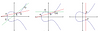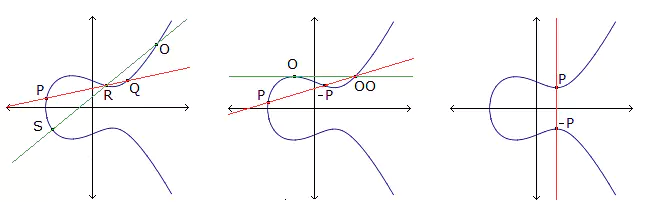# Linear codes from Denniston maximal arcs

### Journal article

D. Bartoli, M. Giulietti, M. Montanucci
Des. Codes Cryptogr., 2017

Semantic Scholar ArXiv DBLP DOI
Cite

### Cite

APA
Bartoli, D., Giulietti, M., & Montanucci, M. (2017). Linear codes from Denniston maximal arcs. Des. Codes Cryptogr.

Chicago/Turabian
Bartoli, D., M. Giulietti, and M. Montanucci. “Linear Codes from Denniston Maximal Arcs.” Des. Codes Cryptogr. (2017).

MLA
Bartoli, D., et al. “Linear Codes from Denniston Maximal Arcs.” Des. Codes Cryptogr., 2017.

## Abstract

In this paper we construct functional codes from Denniston maximal arcs. For $$q=2^{4\ell +2}$$q=24ℓ+2 we obtain linear codes with parameters $$[(\sqrt{q}-1)(q+1),5,d]_q$$[(q-1)(q+1),5,d]q where $$\lim _{q \rightarrow +\infty } d=(\sqrt{q}-1)q-\sqrt{q}$$limq→+∞d=(q-1)q-q. We also find for $$q=16,32$$q=16,32 a number of linear codes which appear to have larger minimum distance with respect to the known codes with same length and dimension.

Share